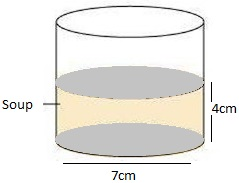Guru

# A patient in a hospital is given soup daily in a cylindrical bowl of diameter 7cm. If the bowl is filled with soup to a height of 4cm, how much soup does the hospital have to prepare daily to serve 250 patients? (Assume π = 22/7) Q.8

• 0

I don’t know how to solve this problem of Surface Areas and Volumes of exercise 13.6 of class 9th math give me the easiest and simple way for solving this tough question of question no.8 A patient in a hospital is given soup daily in a cylindrical bowl of diameter 7cm. If the bowl is filled with soup to a height of 4cm, how much soup does the hospital have to prepare daily to serve 250 patients? (Assume π = 22/7)

Share

1. Diameter of cylindrical bowl = 7 cm

Radius of cylindrical bowl, r = 7/2 cm = 3.5 cm

Bowl is filled with soup to a height of4cm, so h = 4 cmVolume of soup in one bowl= πr2h

(22/7)×3.52×4 = 154

Volume of soup in one bowl is 154 cm3

Therefore,

Volume of soup given to 250 patients = (250×154) cm3= 38500 cm3